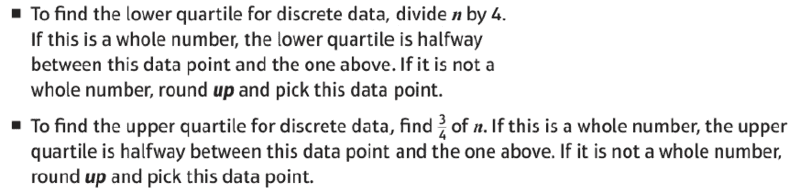# How to calculate Quartiles?

• B
Summary:
Let say I have 21 data, from 1 to 21

What is the upper quartile?
If I do it manually, this is what I do:

1) find the median (which is 11)

2) find the middle data of the "upper data" (data to the right of median), which will be the middle between 16th and 17th data:
$$\frac{16+17}{2}=16.5$$

But I got this note from my teacher:Using that method:
1) find ##\frac 3 4## of n, which is 15.75

2) round up ##\frac{3}{4}n## , which is 16

3) pick 16th data, which is 16

I get two different results. Which one is correct?

Thanks